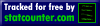﻿ Pythagorean square Numerology meanings | Pythagorean calculator free# Pythagorean square

The famous mathematician Pythagoras believed that the character, behavior and life of man is encoded in the form of numbers where each number has its own character, that is value. If you add two different numbers, you get a third number that will have its significance.

First example
Number 1 is characterized with the courage, and the number 3 is characterized with honesty, and if we add the two numbers 1 + 3 will result in the number 4 and will indicate that a person actions are honest.

Second example
For example, the number 4 is characterized with cunning, and if you add 3 to 4 you get a 7, and would mean that such a person is able to apply the cunning to achieve the goal but it will still be an honest man.

When a child is born two energy are mixed and this cyclical in nature infinite. This is just an example, and in fact these numbers have completely different meaning.

I hope you understand?Example
Date of Birth 25 March 1985 write in the desired cell. Zero is always removed, for example, if the number 30 is necessary to write only 3. Now we fill the square by date of birth.1 We need add up all the the numbers from date of birth. 2 + 5 + 3 + 1 + 9 + 8 + 5 = 332 In order for to fill the second cell, you need to add up the numbers from the first cell 3 + 3 = 63 Now select the number of the first cell and subtract from the second cell and multiply by 2 33-6) x 2 = 544 In order for to fill the fourth cell, add up the numbers from the cell-thirds 5 + 4 = 9Connect the two tables in one and get a table with 8 cellsIt remains to this sort all the numbers.Now you have drawn up a personal Pythagorean square. Each cell has its own quality. Look similarities and compare the Pythagorean square with the table.

## Meaning of numbers on Pythagoras